Advertisement

Advances in Difference Equations

, 2019:341

# An efficient high-order compact finite difference scheme based on proper orthogonal decomposition for the multi-dimensional parabolic equation

Open Access
Research
• 24 Downloads

## Abstract

In this paper, the combination of efficient sixth-order compact finite difference scheme (E-CFDS6) based proper orthogonal decomposition and Strang splitting method (E-CFDS6-SSM) is constructed for the numerical solution of the multi-dimensional parabolic equation (MDPE). For this purpose, we first develop the CFDS6 to attain a high accuracy for the one-dimensional parabolic equation (ODPE). Then, by the Strang splitting method, we have converted the MDPE into a series of one-dimensional ODPEs successfully, which is easier to implement and program with CFDS6 than an alternating direction implicit method. Finally, we employ proper orthogonal decomposition techniques to improve the computational efficiency of CFDS6 and build the E-CFDS6-SSM with fewer unknowns and sufficiently high accuracy. Six numerical examples are presented to demonstrate that the E-CFDS6-SSM not only can largely alleviate the computational load but also hold a high accuracy and simplify the process of the program for the numerical solution of MDPE.

## Keywords

Compact finite difference scheme Proper orthogonal decomposition Multi-dimensional parabolic equation Strang splitting method

## 1 Introduction

Many physical phenomena are simulated with parabolic equations such as proliferation of gas, the penetration of liquids, heat conduction and spread of impurities in semiconductor materials. However, due to the complexity of practical problems or the lack of rules for initial values, their exact solutions for practical engineering problems are not generally sought out so that we have to rely on numerical solutions. Currently, extensive numerical methods including the finite difference method, finite element method, finite volume method and spectral method have been developed for the numerical solution of parabolic equation. In these methods, the FDM has proved to be the most popular and efficient method for finding the numerical solution of a parabolic equation because of its simplicity, wide application in applied fields of sciences and easy programming. Although it is feasible to solve these equations by means of some traditional FDM such as the Euler method and the central difference method, these schemes, which converge very slowly, may largely deviate from the exact solution. Therefore, it is imperative for us to construct a scheme that can guarantee satisfying numerical solution and reflect the properties of equations.

In the existing papers, many scholars focus their eyes on the compact finite difference scheme (CFDS), which has been widely used in numerical solution of various types of partial differential equations. The outstanding advantage of CFDS is that it possesses a faster convergence rate than the corresponding explicit schemes, not significantly increasing the points in each coordinate direction of the grid. As one of the most effective numerical implementations, there have been many numerical research reports concerning with CFDS. For example, Hammad  constructed CFDS for Burgers–Huxley and Burgers–Fisher equations. Wang  developed CFDS for Poisson equation. Mohebbi  combined CFDS with a radial basis functions meshless approach to solve the 2D Rayleigh–Stokes problem. Düring  applied CFDS to stochastic volatility models on non-uniform grids. Chen  provided high-order CFDS to solve parabolic equation. Especially, some attempts have been made whose main idea is to combine fourth Runge–Kutta in time and a sixth-order compact finite difference in space (CFDS6) by the researchers [6, 7, 8]. However, the CFDS6 for parabolic equation, especially the case of desirable accuracy in high dimension, they usually need small spatial discretization or extended finite difference stencils and a small time step which brings about heavy computational loads. Therefore, an important problem for CFDS6 is how to build a scheme which not only saves the computational time in the practical problems but also holds a sufficiently accurate numerical solution.

A large number of numerical examples have proved that the proper orthogonal decomposition (POD) is a powerful technique offering the adequate approximation for numerical models with fewer unknowns, which means that the models based on POD can alleviate the computational loads and guarantee sufficiently accurate solution [9, 10, 11]. The POD is also known as Karhunen–Loève expansions in signal analysis or principal component analysis in statistics, which has been widely used in real-life applications. Especially, it also has been combined with some numerical methods such as finite element methods , meshless methods , finite difference methods  and finite volume methods  successfully. However, to the best of our knowledge, there are no published results in papers concerning efficient CFDS6 (E-CFDS6) for parabolic equations. Thus, the first task in this paper is to build the E-CFDS6 based on the POD method for the parabolic equations.

The MDPE is widely used to describe many physical phenomena, such as engineering problems, typically involving many complex physical phenomena in nature or for irregular computing areas. The study of MDPE has seen a growing interest, which plays an extremely important role in the kinds of fields of physics. Until now, a large amount of work has been done to study FDM with better properties for the numerical solution of MDPE. Among these methods, the alternating direction implicit (ADI) metthod is a popular and well-known method. The main idea of the ADI  method is that the collection of one-dimensional problems can be obtained by the discretization of multi-dimensional problem in space and time, which is feasible since each one-dimensional problem in each time level can be solved by tri-diagonal matrices. Nevertheless, the accuracy of ADI only is of second order, which results in the accumulation of truncated errors in the process of computation. Especially, ADI is more complex to execute as a computer program and it requires a large amount of computational effort, which may produce considerable dissipation. Thus, a crucial issue is how to build more accurate or convenient scheme to solve the multi-dimensional problems. The authors of  introduced a splitting technique whose main idea is to split the Burgers’ equation into two sub-equations and solve them by finite difference schemes. In , the authors have split the full problem into hyperbolic, nonlinear and linear problems, solved by different numerical methods. Sun  had applied a splitting method for solving the radiative transfer problem. Therefore, the Strang splitting method (SSM) is an effective numerical method for solving MDPE by converting MDPE to successions of ODPE. Then we just need to solve the sequence of a linear tri-diagonal system, and the whole process of programming is also simplified. SSM has been applied successfully in a diffusion–reaction problem . To the best of our knowledge, no high-order CFDS combined with POD and SSM (E-CFDS6-SSM) aimed to solve MDPE efficiently has been developed so far. Hence, the second task in this paper is to develop the E-CFDS6-SSM based on the POD and SSM methods to attain a highly accurate numerical solution of MDPE, which only contains very few unknowns and simplifies the whole process of computation.

The outline of this paper is organized as follows. In Sect. 2, a brief background is given on the theoretical foundations of high-order compact finite difference scheme and POD technique. Then the formulation of the CFDS6 is given for ODPE. Besides, the efficient CFDS6 based on POD for solving ODPE is presented. In Sect. 3, the Strang splitting method is described and the E-CFDS6 are extended to MDPE. In Sect. 4, the efficiency, simplicity and capabilities of E-CFDS6-SSM are verified by six numerical examples. The conclusions are drawn in Sect. 5.

## 2 Some high-order difference schemes for ODPE

In this section, we will give a brief description of the CFDS6 and POD techniques, then the construction of E-CFDS6 based on POD for solving ODPE is derived.

### 2.1 The construction of sixth-order compact finite difference scheme for ODPE

Firstly, consider the following initial and boundary value problem:
$$\textstyle\begin{cases} \frac{{\partial u}}{{\partial t}} - a\frac{{{\partial ^{2}}u}}{{\partial{x^{2}}}} = 0, \quad0 < x < L, 0 \le t \le T, \\ u(0,t) = g_{1}(t),\qquad u(L,t) = g_{2}(t), \quad0 \le t \le T, \\ u(x,0) = \varphi(x),\quad0 \le x \le L, \end{cases}$$
(1)
where $$g_{1}(t)$$, $$g_{2}(t)$$ and $$\varphi(x)$$ are given enough smooth functions. Let h be the spatial step increment in the x-direction and τ be the time step increment, and then write $$x_{j} = ( j - 1 )h$$$$(j = 1,2,3, \ldots,J)$$, $$t_{n} = n\tau$$$$(n = 0,1,2, \ldots,N-1)$$, $$u_{j}^{n} \approx u(x_{j},j_{n})$$.

The CFDS can be summarized into two broad categories. The main idea of the first methods is to apply the central difference to the governing partial differential equation and then constantly replace the higher-order derivatives in the truncation error with low-order derivatives of the partial differential equation, which is called the traditional explicit finite difference method. The basic idea in the second methods is that all the spatial derivatives in the governing PDEs can be obtained through solving a system of linear equations [21, 22, 23]. In this paper, we choose the second way to build a high-order compact finite difference scheme for a parabolic equation.

Because the parabolic equation (1) containing the second-order derivatives, we only give the compact finite difference scheme for second-order derivatives. Next, we derive the CFDS6 for an ODPE. For the second-order derivatives at interior nodes $$u_{j}$$, the sixth-order scheme formula can be written as follows:
$$\frac{2}{{11}}u''_{j - 1} + u''_{j} + \frac{2}{{11}}u''_{j + 1} = \frac{3}{{11{h^{2}}}} \biggl( \frac{{{u_{j - 2}} + 16{u_{j - 1}}}}{4} - \frac{{17}}{2}u_{j} + \frac{{{u_{j + 2}} + 16{u_{j + 1}}}}{4} \biggr).$$
(2)
At the most left boundary point $$x_{1}$$, a sixth-order formula can be given as follows:
\begin{aligned}[b] &u''_{1} + \frac{{126}}{{11}}u''_{2} \\ &\quad = \frac{1}{{{h^{2}}}} \biggl( \frac{{2077}}{{157}}{u_{1}} - \frac{{2943}}{{110}}{u_{2}} + \frac{{573}}{{44}}{u_{3}} + \frac {{167}}{{99}}{u_{4}} - \frac{{18}}{{11}}{u_{5}} + \frac{{57}}{{110}}{u_{6}} - \frac{{131}}{{1980}}{u_{7}} \biggr). \end{aligned}
(3)
At the second left boundary point $${x_{2}}$$, the sixth-order formula is given as follows:
\begin{aligned}[b] &\frac{{11}}{{128}}{u''_{1}} + {u''_{2}} + \frac{{11}}{{128}}{u''_{3}} \\ &\quad = \frac{1}{{{h^{2}}}} \biggl( \frac{{585}}{{512}}{u_{1}} - \frac {{141}}{{64}}{u_{2}} + \frac{{585}}{{512}}{u_{3}} + \frac{9}{{32}}{u_{4}} - \frac{{81}}{{512}}{u_{5}} + \frac {3}{{64}}{u_{6}} - \frac{3}{{512}}{u_{7}} \biggr). \end{aligned}
(4)
According to the symmetry, at the second right boundary point $${x_{J - 1}}$$, the sixth-order formula is
\begin{aligned}[b] & \frac{{11}}{{128}} {u''_{J}} + {u''_{J - 1}} + \frac {{11}}{{128}}{u''_{J - 2}} \\ & \quad = \frac{1}{{{h^{2}}}} \biggl( \frac{{585}}{{512}}{u_{J}} - \frac {{141}}{{64}}{u_{J - 1}} + \frac{{585}}{{512}}{u_{J - 2}} + \frac{9}{{32}}{u_{J - 3}} \\ &\qquad {} - \frac{{81}}{{512}}{u_{J- 4}} + \frac {3}{{64}}{u_{J - 5}} - \frac{3}{{512}}{u_{J - 6}} \biggr). \end{aligned}
(5)
Similarly, at the most right boundary point $${x_{J}}$$, a sixth-order formula is
\begin{aligned}[b] &{u''_{J - 1}} + {u''_{J}} \\ &\quad = \frac{1}{{{h^{2}}}} \biggl( \frac{{2077}}{{157}}{u_{J}} - \frac {{2943}}{{110}}{u_{J - 1}} + \frac{{573}}{{44}}{u_{J - 2}} + \frac{{167}}{{99}}{u_{J - 3}} \\ &\qquad {} - \frac{{18}}{{11}}{u_{J - 4}} + \frac{{57}}{{110}}{u_{J - 5}} - \frac {{131}}{{1980}}{u_{J - 6}} \biggr). \end{aligned}
(6)
Note that the scheme of Eqs. (2)–(6) can be written as
$$\mathbf{{AU}}'' = \mathbf{{BU}},$$
(7)
where
$\begin{array}{c}\mathbf{U}={\left({u}_{1},{u}_{2},\dots ,{u}_{J-1},{u}_{J}\right)}^{T},\hfill \\ \mathbf{A}={\left[\begin{array}{ccccccc}1& \frac{126}{11}& & & & & \\ \frac{11}{128}& 1& \frac{11}{128}& & & & \\ & \frac{2}{11}& 1& \frac{2}{11}& & & \\ & & \ddots & \ddots & \ddots & & \\ & & & \frac{2}{11}& 1& \frac{2}{11}& \\ & & & & \frac{11}{128}& 1& \frac{11}{128}\\ & & & & & \frac{126}{11}& 1\end{array}\right]}_{J×J},\hfill \\ \mathbf{B}=\frac{1}{a{h}^{2}}{\left[\begin{array}{cccccccc}\frac{2077}{157}& -\frac{2943}{110}& \frac{574}{44}& \frac{167}{99}& -\frac{18}{11}& \frac{57}{110}& -\frac{131}{1980}& \\ \frac{585}{512}& -\frac{141}{64}& \frac{459}{512}& \frac{9}{32}& -\frac{81}{512}& \frac{3}{64}& -\frac{3}{512}& \\ \frac{3}{44}& \frac{12}{11}& -\frac{51}{22}& \frac{12}{11}& \frac{3}{44}& & & \\ & \ddots & \ddots & \ddots & \ddots & \ddots & & \\ & & \frac{3}{44}& \frac{12}{11}& -\frac{51}{22}& \frac{12}{11}& \frac{3}{44}& \\ & -\frac{3}{512}& \frac{3}{64}& -\frac{81}{512}& \frac{9}{32}& \frac{459}{512}& -\frac{141}{64}& \frac{585}{512}\\ & -\frac{131}{1980}& \frac{57}{110}& -\frac{18}{11}& \frac{167}{99}& \frac{574}{44}& -\frac{2943}{110}& \frac{2077}{157}\end{array}\right]}_{J×J}.\hfill \end{array}$
As mentioned above, the parabolic equation in (1) has been converted into a system of initial value problem of ordinary differential equations (ODEs) by the compact scheme (2)–(6). Then the fourth-order Runge–Kutta (RK4) scheme is applied to integrate the time-dependent governing ODEs,
$$\frac{{d\mathbf {U}}}{{dt}} = R(\mathbf {U}),$$
(8)
where R denotes a spatial differential operator. Assuming that the value of $$\mathbf {U}^{n}$$ at $${t_{n}}$$ is given, then the numerical solution $$\mathbf {U}^{n + 1}$$ at $${t_{n + 1}} = {t_{n}} + \tau$$ is obtained through the following operations:
$$\textstyle\begin{cases} \mathbf {k}_{0} = \tau\cdot R(\mathbf {U}^{n}),\qquad \mathbf {U}_{1} = \mathbf {U}^{n} + \mathbf {k}_{0}/2, \\ \mathbf {k}_{1} = \tau\cdot R(\mathbf {U}_{1}),\qquad \mathbf {U}_{2} = \mathbf {U}^{n} + \mathbf {k}_{1}/2, \\ \mathbf {k}_{2} = \tau\cdot R(\mathbf {U}_{2}),\qquad \mathbf {U}_{3} = \mathbf {U}^{n} + \mathbf {k}_{2}, \\ \mathbf {k}_{3} = \tau\cdot R(\mathbf {U}_{3}),\qquad \mathbf {K}=\mathbf {k}_{0} + 2\mathbf {k}_{1} + 2\mathbf {k}_{2} + \mathbf {k}_{3}, \\ \mathbf {U}^{n + 1} = \mathbf {U}^{n} + \frac{1}{6}\mathbf {K}. \end{cases}$$
(9)
Using the sixth-order compact difference scheme listed in Eq. (7), the second derivative related to the operator $$R(\mathbf {U})$$ at each time level is obtained. Then we can get the numerical solution at $${t_{n + 1}}$$ by the RK4 method. Thus, if the initial value is known, we can calculate the value at any time steps through many iterations.

### 2.2 The establishment of E-CFDS6 based on POD technique

In this section, we use the POD technique to build the E-CFDS6. For more details, not described here, please refer to [15, 24, 25, 26]. Meanwhile, the POD method has a variety of interpretations, refer to [9, 10, 11] to find more interpretations. As described in the Introduction, the main goal of POD is to seek a set of orthogonal matrices generated by applying a singular value decomposition (SVD) into sample space, which is called an optimal basis function. Then, by using the first M sequences of the optimal basis function, the samples can be expressed optimally. In this method, POD will be used to calculate the optimal basis function. To this aim, we need a set of snapshots and use SVD to construct the optimal basis.

We suppose that there are d samples (also usually called snapshots) $${\mathbf{{s}}^{{{1}}}},{\mathbf{{s}}^{{{2}}}}, \ldots , {\mathbf{{s}}^{{{d}}}}$$ which can be written as a matrix $$\mathbf{{S}} = ({\mathbf{{s}}^{1}},{\mathbf{{s}}^{2}}, \ldots ,{\mathbf{{s}} ^{d}})$$, where $$\mathbf{{s}}^{i} \in{R^{J}}$$$$(i = 1,2, \ldots,d)$$. $$\mathbf{{S}} \in{R^{J \times d}}$$, and $$\mathbf{{S}}{\mathbf{{S}} ^{T}} \in{R^{J \times J}}$$ is an $$J \times J$$ semi-definite matrix. Applying the SVD on matrix S:
$\mathbf{S}=\mathbf{G}\left[\begin{array}{cc}{\mathbf{D}}_{r}& 0\\ 0& 0\end{array}\right]{\mathbf{V}}^{T},$
(10)
the matrix $$\mathbf {G} = (\boldsymbol{\alpha}_{1},\boldsymbol{\alpha}_{2}, \ldots, \boldsymbol{\alpha}_{J})$$ with J rows and J columns. The G and $${\mathbf {V}_{d \times d}}$$ are both orthogonal matrices, $$\mathbf {D}_{r} = \operatorname{diag}({\lambda_{1}},{\lambda_{2}}, \ldots,{\lambda_{r}})$$. The orthogonal eigenvectors of $$\mathbf {S}{\mathbf {S} ^{T}}$$ are contained in the matrix $$\mathbf {G} = (\boldsymbol{\alpha}_{1}, \boldsymbol{\alpha}_{2}, \ldots,\boldsymbol{\alpha}_{J})$$. The singular values $${\lambda_{i}}$$$$(i = 1,2, \ldots,r)$$ satisfy $${\lambda_{1}} \ge {\lambda_{2}} \ge\cdots\ge{\lambda_{r}} > 0$$.
Denote d columns of S by $${\boldsymbol{\beta}^{l}} = {(s_{1}^{l},s _{2}^{l}, \ldots,s_{J}^{l})^{T}}$$$$(l = 1,2, \ldots,d)$$, the projection $${P_{k}}$$ is defined as follows:
$${P_{M}}\bigl(\boldsymbol{\beta}^{l}\bigr) = \sum_{i = 1}^{M} {\bigl({\boldsymbol{\alpha} _{i}},{\boldsymbol{\beta}^{l}}\bigr)} {\boldsymbol{\alpha}_{i}},$$
(11)
the $$0 < M \le d$$ and $$( \cdot, \cdot)$$ represents the inner product of vectors, then we can obtain the following result :
$$\bigl\Vert {\boldsymbol{\beta}^{l}} - {P_{M}}\bigl({\boldsymbol{\beta}^{l}}\bigr) \bigr\Vert _{{2}} \le{\lambda _{M + 1}},$$
(12)
where $$\Vert \cdot\Vert_{{2}}$$ is standard norm of vector. Hence, $${\boldsymbol{\alpha}_{1}},{\boldsymbol{\alpha}_{2}}, \ldots,\boldsymbol{\alpha}_{M}$$ are a group of the optimal POD basis, which from basis matrix $$\boldsymbol{\alpha} = (\boldsymbol{\alpha}_{1},\boldsymbol{\alpha}_{2}, \ldots, \boldsymbol{\alpha}_{M})$$. It should be pointed out that the basis matrix fulfills the orthogonality condition, i.e., $${{\boldsymbol{\alpha}}^{T}} {\boldsymbol{\alpha}} = \mathbf {I}$$ (I is unit matrix with M dimension).

In the following, the procedure of establishing E-CFDS6 for parabolic equation is listed by the POD basis.

If U of Eq. (7) is substituted for
$$\mathbf {U}^{*} = \boldsymbol{\alpha} \mathbf {V} = {\boldsymbol{\alpha} _{J \times M}} {\mathbf {V}_{M \times1}},$$
(13)
we have
$${\mathbf {V}}^{\prime\prime} = {{\boldsymbol{\alpha}}^{T}} { \mathbf {A}^{ - 1}} {\mathbf {B}\boldsymbol{\alpha}} \mathbf {V},$$
(14)
and noting that $${{\boldsymbol{\alpha}}^{T}}{\boldsymbol{\alpha}} = \mathbf {I}$$, let $${\mathbf {V}_{\mathbf{{0}}}}= {\boldsymbol{\alpha} ^{T}}{\mathbf{{U}}^{n}}$$ then the RK4 for the reduced solution is given as follows:
$$\textstyle\begin{cases} {\overline{\mathbf {k}} _{0}} = \tau\cdot R({\mathbf {V}_{0}}),\qquad{\mathbf {V}_{1}} = {\mathbf {V}_{0}} + {\overline{\mathbf {k}} _{0}}/2, \\ {\overline{\mathbf {k}} _{1}} = \tau\cdot R({\mathbf {V}_{1}}),\qquad {\mathbf {V}_{2}} = {\mathbf {V}_{0}} + {\overline{\mathbf {k}} _{1}}/2, \\ {\overline{\mathbf {k}} _{2}} = \tau\cdot R({\mathbf {V}_{2}}),\qquad {\mathbf {V}_{3}} = {\mathbf {V}_{0}} + {\overline{\mathbf {k}} _{2}}, \\ {\overline{\mathbf {k}} _{3}} = \tau\cdot R({\mathbf {V}_{3}}),\qquad \overline{\mathbf {K}}={\overline{\mathbf {k}} _{0}} + 2{\overline{\mathbf {k}}_{1}} + {\overline{\mathbf {k}} _{2}} + {\overline{\mathbf {k}} _{3}}, \\ {\mathbf {V}^{n + 1}} = {\mathbf {V}_{\mathbf{{0}}}} + \frac{1}{6}\overline{\mathbf {K}}. \end{cases}$$
(15)
We can obtain the global solution $${\mathbf {U}^{n + 1}} = {\boldsymbol{\alpha}} {\mathbf {V}^{n + 1}}$$ when the reduced solution $${\mathbf {V}^{n + 1}}$$ has been obtained from Eq. (15). Here, the procedure of E-CFDS6 for parabolic equation is listed as follows:
1. step 1

Snapshot S is generated from experiments or numerical simulations.

2. step 2

Formulate the optimal POD basis matrix α by the SVD method.

3. step 3

Apply Eq. (14) to work out the reduced second-order derivative $$\mathbf {V}''$$.

4. step 4

Obtain the reduced solution by solving Eq. (15).

5. step 5

Having applied $${\mathbf {U}^{n + 1}} = {\boldsymbol{\alpha}}{ \mathbf {V}^{n + 1}}$$, then the reduced solution is expanded.

It is easy to see from that above algorithm that E-CFDS6 needs to solve only $$M \times M$$ equations (Eq. (14)) at each iteration, but CFDS6 includes $$J \times J$$ equations (Eq. (7)) to solve at each iteration. In general, M is much smaller than J, which means that E-CFDS6 needs less computational time than CFDS6. Applying that whole procedure, we may complete the entire calculation from $${t^{n}}$$ to $${t^{n + 1}}$$. Moreover, due to the use of a sixth-order compact scheme for discretizing the space variables, it is not difficult to find that our algorithm is of sixth-order accuracy.

## 3 Multi-dimensional case

The traditional numerical method for solving MDPE is the ADI method, which replaces complex multi-dimensional problems with a number of one-dimensional problems. It is a classical algorithm. However, with the use of the Crank–Nicolson method in time and the center difference method in space, ADI is shown to only have second-order accuracy in both time and space. Besides, this method produces a very complex set of equations in MDPE, which is very expensive to solve. The benefits of the ADI method are that the equation needed to be solved in each step is relatively simple, and the tri-diagonal matrix algorithm can be used to solve the equation successfully. The ADI method is unconditionally stable. Compared with ADI, SSM is an effective numerical method that will lead to no loss of accuracy. SSM is extremely efficient for solving MDPE by converting MDPE to a product of ODPE and programming is very simple. It also can be use to accelerate that calculation of problems related to operators of different time scales. In this section, instead of using ADI, we applied E-CFDS6-SSM to solve MDPE. First, we apply SSM to decompose MDPE into a product of ODPEs. Then we solve each ODPE by E-CFDS6.

### 3.1 Solutions to two-dimensional parabolic equation

Considering the following two-dimensional parabolic equation:
$$\frac{{\partial u}}{{\partial t}} = b \biggl( \frac{{{\partial ^{2}}u}}{{\partial{x^{2}}}} + \frac{{{\partial^{2}}u}}{{\partial{y^{2}}}} \biggr), \quad (x,y,t) \in\varOmega\times[0,T].$$
(16)
We rewrite Eq. (16) as follows:
$$\textstyle\begin{cases} \frac{{\partial{u_{1}}}}{{\partial t}} = b\frac{{{\partial ^{2}}{u_{1}}}}{{\partial{x^{2}}}}, \\ \frac{{\partial{u_{2}}}}{{\partial t}} = b\frac{{{\partial ^{2}}{u_{2}}}}{{\partial{y^{2}}}}, \end{cases}$$
(17)
and
$$\textstyle\begin{cases} \frac{{d\mathbf {U}_{1}}}{{d t}} = {\mathbf {H}_{x}}\mathbf {U}_{1}, \\ \frac{{d\mathbf {U}_{2}}}{{d t}} = {\mathbf {H}_{y}}\mathbf {U}_{2}, \end{cases}$$
(18)
where $${\mathbf {H}_{x}}$$ and $${\mathbf {H}_{y}}$$ are different operators solved by Eq. (7) in the x-direction and the y-direction. The initial value of 2D equation $${\mathbf {U}^{n }}$$ at $${t^{n }}$$ is split, respectively, into $$\mathbf {U}_{1}^{n }$$ and $$\mathbf {U}_{2}^{n }$$ in the x-direction and the y-direction by Eq. (17). Then we use E-CFDS6 to compute $$\mathbf {U}_{1}^{n + 1}$$ and $$\mathbf {U}_{2}^{n + 1}$$ by Eq. (15). Finally, we obtain the following equations:
$${\mathbf {U}^{n + 1}} = \mathbf {U}_{1}^{n + 1} \cdot{\bigl(\mathbf {U}_{2}^{n + 1}\bigr)^{T}},$$
(19)
where $${\mathbf {U}^{n + 1}}$$ represents the numerical result of 2D equation at $${t^{n+1}}$$. Thus, we split the two-dimensional problem into two one-dimensional problems, which indicates that we only solve each ODPE by E-CFDS6 introduced in Sect. 2, rather than solve a set of complicated equations.

### 3.2 Solutions to three-dimensional parabolic equation

For the three-dimensional parabolic equation, the above E-CFDS6-SSM scheme can be extended directly to the three-dimensional case. Similarly, consider the following three-dimensional parabolic equation:
$$\frac{{\partial u}}{{\partial t}} = c \biggl( \frac {{{\partial^{2}}u}}{{\partial{x^{2}}}} + \frac{{{\partial^{2}}u}}{{\partial{y^{2}}}} + \frac{{{\partial ^{2}}u}}{{\partial{z^{2}}}} \biggr), \quad(x,y,z,t) \in\varOmega \times[0,T].$$
(20)
We also rewrite Eq. (20) as follows:
$$\textstyle\begin{cases} \frac{{\partial{u_{1}}}}{{\partial t}} = c\frac{{{\partial ^{2}}{u_{1}}}}{{\partial{x^{2}}}}, \\ \frac{{\partial{u_{2}}}}{{\partial t}} = c\frac{{{\partial ^{2}}{u_{2}}}}{{\partial{y^{2}}}}, \\ \frac{{\partial{u_{3}}}}{{\partial t}} = c\frac{{{\partial ^{2}}{u_{3}}}}{{\partial{z^{2}}}}, \end{cases}$$
(21)
and
$$\textstyle\begin{cases} \frac{{d\mathbf {U}_{1}}}{{dt}} = {\mathbf {H}_{x}}\mathbf {U}_{1}, \\ \frac{{d\mathbf {U}_{2}}}{{dt}} = {\mathbf {H}_{y}}\mathbf {U}_{2}, \\ \frac{{d\mathbf {U}_{3}}}{{dt}} = {\mathbf {H}_{z}}\mathbf {U}_{3}, \end{cases}$$
(22)
where $${\mathbf {H}_{x}}$$, $${\mathbf {H}_{y}}$$, $${\mathbf {H}_{z}}$$ are different operators obtained by Eq. (7) in the x-direction, y-direction, and z-direction. It is necessary to note that the way of acquiring $${\mathbf {U}^{n + 1}}$$ is similar to Eq. (19), which will not be listed again.

The $$\mathbf {U}_{1}^{n + 1}$$, $$\mathbf {U}_{2}^{n + 1}$$ and $$\mathbf {U}_{3}^{n + 1}$$ represent the numerical results of each ODPE obtained by Eq. (15) in the x-direction, y-direction and z-direction. Compared with ADI, it is so simple that we do not solve a very complicated set of equations in MDPE in the way mentioned for ADI, and we just need to compute three ODPEs, respectively. It should be pointed out that the scheme has the same accuracy as the one-dimensional cases.

### 3.3 Formulate POD basis for each ODPE split by MDPE

In this subsection, we will illustrate our methods and formulate the POD basis for each ODPE. As described above, we have split 2D or 3D parabolic equations into a series of ODPE. By solving the formulation of Eq. (15), we can get the approximate solutions $$\{ {u_{j}^{n}} \}_{n = 1}^{N}$$$$( j = 2, 3, \ldots,J - 1 )$$. Then we may select $$\{ {u_{j}^{{n_{i}}}} \}_{i = 1}^{d}$$$$( j = 2, 3, \ldots,J - 1, 1 \le{n_{1}} \le {n_{2}} < \cdots< n_{{{d}}} \le N )$$ from the CFDS6 solutions $$\{ {u_{j}^{n}} \}_{n = 1}^{N}$$$$( j = 2, 3, \ldots,J - 1 )$$ of Eq. (15), which is called the method of ‘snapshots’. Finally, we can construct the POD basis for each ODPE in the way mentioned in Eqs. (10)–(13).

Although the snapshot is obtained through the approximate solution of CFDS6 in this paper, in fact, we can get the collection of snapshots through experiments and interpolation when calculating the actual problem. If the development and change of a large number of future natural phenomena (for example, weather change, biology anagenesis, and so on) are closely related to previous results, or if the physical system of the natural phenomena performs well, that is, the past dynamics is representative and inclusive of the future dynamics, then the previous or existing experimental data can be used to construct a snapshot. Then the POD basis is obtained by using the POD method in Eqs. (10)–(13) and we can derive an efficient scheme. Therefore, one can effectively simulate and predict the development and change of some future natural phenomena, which is of great significance for practical applications.

## 4 Numerical examples

In order to see whether the present method is capable of getting an accurate solution, in this section, the E-CFDS6-SSM will be evaluated for six examples of the MDPE given below. In the case of the different number of nodes, we have some tests of the accuracy and efficiency for the method described in this article. We performed our computations using Matlab 2018a software on a Ryzen 7 1800X, 3.6 GHz CPU machine with 16 GB of memory. The convergence order of the method presented in this article is calculated with this formula:
$$R = \frac{{\log\frac{{\mathit{error}_{\mathrm{new}}}}{ {\mathit{error}_{\mathrm{old}}}}}}{{\log\frac{{{h_{\mathrm{new}}}}}{ {{h_{\mathrm{old}}}}}}}.$$

### Example 1

Consider the following 2D parabolic equation ($$\mathrm{SP} _{1}$$):
$$\textstyle\begin{cases} \frac{{\partial u}}{{\partial t}} = \frac{{{\partial ^{2}}u}}{{\partial{x^{2}}}} + \frac{{{\partial^{2}}u}}{{\partial{y^{2}}}}, \quad (x,y) \in\varOmega, 0 < t \le1, \\ u(x,y,0) = {10^{9}}\sin\pi x\sin\pi y, \quad (x,y) \in\varOmega, \\ u(x,y,t) = 0,\quad (x,y) \in\partial\varOmega, 0 < t \le1, \end{cases}$$
where $$\varOmega= \{ (x,y);0 \le x \le2,0 \le y \le2\}$$, ∂Ω denotes the boundary of Ω. The exact solution is $$u(x,y,t) = {10^{9}}{e^{ - 2{\pi^{2}}t}}\sin\pi x\sin\pi y$$.
The obtained solutions and point-wise error of CFDS6-SSM with $$41\times 41$$ uniformly distributed points are shown in Fig. 1 while the corresponding numerical results of E-CFDS6-SSM are shown in Fig. 2. We choose h as 0.05 and $$\tau=0.0001$$. It is not difficult to see that the results of E-CFDS6-SSM are in very good agreement with those of CFDS6-SSM, which means that the E-CFDS6-SSM method possesses a high computational accuracy, as well as CFDS6-SSM. We compare the error of E-CFDS6-SSM and CFDS6-SSM with the error of the D’Yakonov alternating direction implicit (DADI) method with $${h_{x}} = {h_{y}} = 0.01$$ and $$\tau= 0.01$$ in Table 1, which indicates that our algorithm significantly improves the accuracy. In order to compare the accuracy and efficiency of two approximate methods, we list the error and computational time of E-CFDS6-SSM and CFDS6-SSM in Table 1 and Table 2. The error of CFDS6-SSM and the error of E-CFDS6-SSM are also drawn in Figs. 1 and 2. It also can be clearly seen that the E-CFDS6-SSM is almost as accurate as CFDS6-SSM and the computational times of E-CFDS6-SSM are less than those of CFDS6-SSM under the same number of nodes. In Fig. 3, the error between CFDS6-SSM and E-CFDS6-SSM is no more than $$3 \times 10^{ - 7}$$. It also can be found that the accuracy of E-CFDS6-SSM is almost identical to that of CFDS6-SSM. Besides, we also found that the order of convergence obtained by E-CFDS6-SSM and CFDS6-SSM is almost the same in Table 2. In addition, it should be noted that our algorithm is easier to execute than the classical ADI algorithm.Figure 1 The CFDS6-SSM solution (left-hand side) and error of CFDS6-SSM (right-hand side) when $$J=41$$ and $$t=1$$ for $$\mathrm{SP} _{1}$$Figure 2 The E-CFDS6-SSM solution (left-hand side) and error of E-CFDS6-SSM (right-hand side) when $$J =41$$ and $$t=1$$ for $$\mathrm{SP} _{1}$$Figure 3 The error between the solution of CFDS6-SSM and solution of E-CFDS6-SSM when $$J = 41$$ and $$t=1$$ for $$\mathrm{SP} _{1}$$
Table 1

The error of two different schemes for $$\mathrm{SP} _{1}$$ at $$t=1$$

$$({x_{i}},{y_{i}})$$

CFDS6-SSM

E-CFDS6-SSM

DADI (error)

(0.4, 0.4)

1.0284e−06

1.2736e−06

1.5021e−02

(0.8, 0.8)

3.9282e−07

4.7769e−07

5.8051e−03

(1.2, 1.2)

3.9282e−07

4.7769e−07

5.5856e−03

(1.6, 1.6)

1.0284e−06

1.2736e−06

1.4801e−02

Nodes

41 × 41

41 × 41

201 × 201

Table 2

The convergence order and computational time for $$\mathrm {SP} _{1}$$ at $$t=1$$

Nodes

11 × 11

21 × 21

41 × 41

CFDS6-SSM

Max (error)

0.0441

2.1770e−04

1.3977e−06

Time (s)

10.099649

126.587866

416.107510

Order

7.662

7.283

E-CFDS6-SSM

Max (error)

0.0197

5.9319e−05

1.1370e−06

Time (s)

1.091099

51.221890

234.497833

Order

8.375

5.705

### Example 2

Consider the following 2D parabolic equation ($$\mathrm{SP} _{2}$$):
$$\textstyle\begin{cases} ( 1 + \frac{1}{{{2^{2}}}} )\frac{{\partial u}}{{\partial t}} = \frac{{{\partial^{2}}u}}{{\partial{x^{2}}}} + \frac{{{\partial ^{2}}u}}{{\partial{y^{2}}}},\quad (x,y) \in\varOmega, 0 < t \le2, \\ u(x,y,0) = \sin x\sin\frac{y}{2},\quad (x,y) \in\varOmega, \\ u(x,y,t) = 0,\quad (x,y) \in\partial\varOmega, 0 < t \le2, \end{cases}$$
where $$\varOmega= \{ (x,y);0 \le x \le2\pi,0 \le y \le2\pi\}$$, ∂Ω denotes the boundary of Ω. The exact solution is $$u(x,y,t) = {e^{ - t}}\sin x\sin\frac{y}{2}$$.
We design this equation to let the solution u change much faster in the x-direction than in y-direction. We give the figures of CFDS6-SSM and E-CFDS6-SSM in Figs. 4 and 5. Obviously, they are very similar for the same nodes. Meanwhile, we also plot the error of E-CFDS6-SSM and CFDS6-SSM in the right-hand side of Figs. 4 and 5 for comparison. The error of CFDS6-SSM, the error of E-CFDS6-SSM and the computational time are also shown in Table 3 and Table 4. It is not difficult to see that the results of E-CFDS6-SSM are in very good agreement with those of CFDS6-SSM, which means that the E-CFDS6-SSM not only possesses a high accuracy as well as CFDS6-SSM, but also alleviates computational load. In addition, we compare the error of E-CFDS6-SSM and CFDS6-SSM with the error of DADI with $${h_{x}} = {h_{y}} = 0.01\pi$$ and $$\tau= 0.01$$ in Table 3, it also manifested that our algorithm significantly improves the accuracy. In Fig. 6, the error between CFDS6-SSM and E-CFDS6-SSM is no more than $$1.5 \times10^{ - 9}$$. It also indicated that the accuracy of E-CFDS6-SSM is almost identical to that of CFDS6-SSM under the same nodes and time step. Besides, we also give the order of convergence obtained by E-CFDS6-SSM and CFDS6-SSM in Table 4, by which it can be seen clearly that the numerical results confirm the convergence with the rate $$O({h^{6}})$$ for this equation.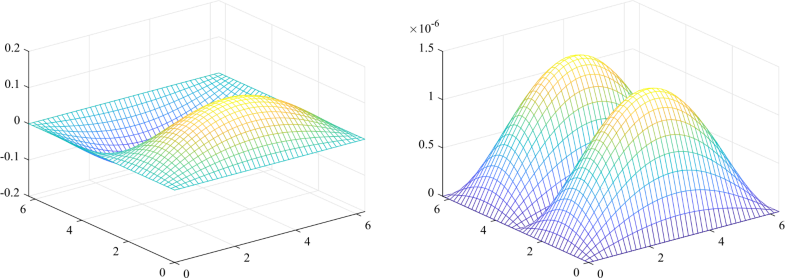Figure 4 The CFDS6-SSM solution (left-hand side) and error of CFDS6-SSM (right-hand side) when $$J=41$$ and $$t=2$$ for $$\mathrm{SP} _{2}$$Figure 5 The E-CFDS6-SSM solution (left-hand side) and error of E-CFDS6-SSM (right-hand side) when $$J=41$$ and $$t=2$$ for $$\mathrm{SP} _{2}$$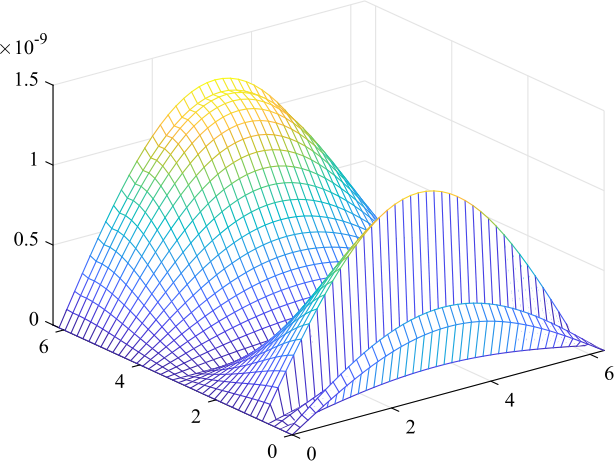Figure 6 The error between the solution of CFDS6-SSM and solution of E-CFDS6-SSM when $$J=41$$ and $$t=2$$ for $$\mathrm{SP} _{2}$$
Table 3

The error of two different schemes for $$\mathrm{SP} _{2}$$ at $$t=2$$

$$({x_{i}},{y_{i}})$$

CFDS6-SSM

E-CFDS6-SSM

DADI (error)

(0.2π, 0.2π)

1.3405e−06

1.3405e−06

8.4424e−5

(0.8π, 0.8π)

4.1228e−06

4.1230e−06

2.5983e−4

(1.2π, 1.2π)

4.1228e−06

4.1230e−06

2.5982e−4

(1.8π, 1.8π)

1.3396e−06

1.3405e−06

8.4416e−5

Nodes

41 × 41

41 × 41

201 × 201

Table 4

The convergence order and computational time for $$\mathrm {SP} _{2}$$ at $$t=2$$

Nodes

21 × 21

41 × 41

81 × 81

CFDS6-SSM

Max (error)

1.7206e−04

1.3604e−06

2.5087e−08

Time (s)

24.018882

52.936624

121.629286

Order

6.982

5.761

E-CFDS6-SSM

Max (error)

1.7280e−04

1.3614e−06

2.5088e−08

Time (s)

5.014861

12.554003

32.825120

Order

6.988

5.762

### Example 3

Consider the following 2D parabolic equation ($$\mathrm{SP} _{3}$$):
$$\textstyle\begin{cases} \frac{{\partial u}}{{\partial t}} - (\frac{{{\partial ^{2}}u}}{{\partial{x^{2}}}} + \frac{{{\partial^{2}}u}}{{\partial{y^{2}}}}) = {e^{ - t}}\sin x\sin y,\quad 0 < t \le2, \\ u(x,y,0) = \sin x\sin y,\quad (x,y) \in\varOmega, \\ u(x,y,t) = 0,\quad (x,y) \in\partial\varOmega, 0 < t \le2, \end{cases}$$
where $$\varOmega= \{ (x,y);0 \le x \le\pi,0 \le y \le\pi\}$$, ∂Ω denotes the boundary of Ω. The exact solution is $$u(x,y,t) = {e^{ - t}}\sin x\sin y$$.
Figure 7 and Fig. 8 plot the numerical solutions and point-wise absolute errors of CFDS6-SSM and E-CFDS6-SSM, respectively. For comparison, Table 5 lists the error of E-CFDS6-SSM and DADI with $${h_{x}} = {h_{y}} = 0.005\pi$$ and $$\tau= 0.01$$, which indicates that our methods remarkably improve the accuracy. Similarly, we also list the absolute error, computational time and convergence order in Table 6. It can be clearly seen that the E-CFDS6-SSM greatly saved the time consumption and vastly improved the computational efficiency compared with CFDS6-SSM. Besides, the convergence order in Table 6 confirms the convergence with the rate $$O({h^{6}})$$ for this equation. The error between CFDS6-SSM and E-CFDS6-SSM is also drawn in Fig. 9. It also can be clearly seen that the CFDS6-SSM is almost accurate with the E-CFDS6-SSM.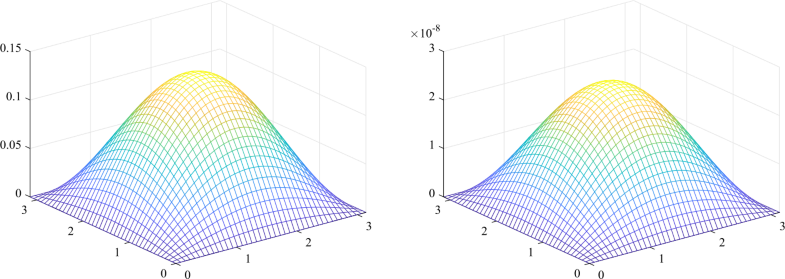Figure 7 The figure of CFDS6-SSM solution (left-hand side) and error of CFDS6-SSM (right-hand side) when $$J=41$$ and $$t=2$$ for $$\mathrm{SP} _{3}$$Figure 8 The E-CFDS6-SSM solution (left-hand side) and error of E-CFDS6-SSM (right-hand side) when $$J=41$$ and $$t=2$$ for $$\mathrm{SP} _{3}$$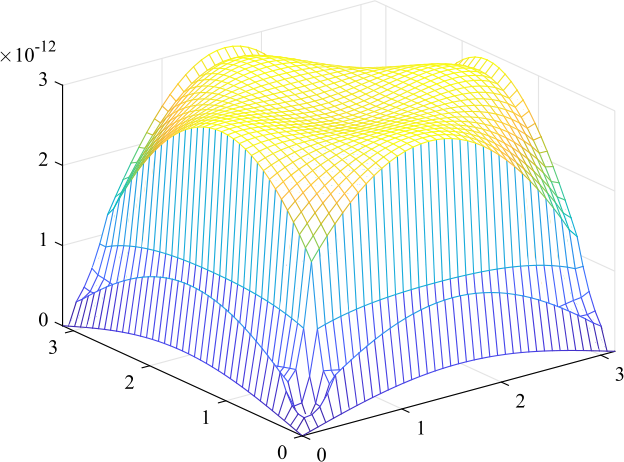Figure 9 The error between the solution of CFDS6-SSM and solution of E-CFDS6-SSM when $$J=41$$ and $$t=2$$ for $$\mathrm{SP} _{3}$$
Table 5

The error of two different schemes for $$\mathrm{SP} _{3}$$ at $$t=2$$

$$({x_{i}},{y_{i}})$$

CFDS6-SSM

E-CFDS6-SSM

DADI (error)

(0.2π, 0.2π)

8.6691e−09

8.6716e−09

9.0752e−04

(0.4π, 0.4π)

2.2696e−08

2.2699e−08

2.3615e−03

(0.6π, 0.6π)

2.2696e−08

2.2699e−08

2.3437e−03

(0.8π, 0.8π)

8.6691e−09

8.6716e−09

8.8970e−04

Nodes

41 × 41

41 × 41

201 × 201

Table 6

The convergence order and computational time for $$\mathrm {SP} _{3}$$ at $$t=2$$

Nodes

11 × 11

21 × 21

41 × 41

CFDS6-SSM

Max (error)

1.7091e−04

1.3605e−06

2.5092e−08

Time (s)

1.008504

24.672396

56.174196

Order

6.972

5.761

E-CFDS6-SSM

Max (error)

1.7344e−04

1.3630e−06

2.5095e−08

Time (s)

0.525925

11.531699

31.044471

Order

6.991

5.763

### Example 4

Consider the following 3D parabolic equation ($$\mathrm{SP} _{4}$$):
$$\textstyle\begin{cases} ( 1 + \frac{1}{{{2^{2}}}} + 2^{2} )\frac{{\partial u}}{{\partial t}} = \frac{{{\partial^{2}}u}}{{\partial{x^{2}}}} + \frac{{{\partial ^{2}}u}}{{\partial{y^{2}}}} + \frac{{{\partial^{2}}u}}{{\partial{z^{2}}}},\quad (x,y,z) \in\varOmega , 0 < t \le2, \\ u(x,y,z,0) = \sin x\sin\frac{y}{2}\sin2z,\quad (x,y,z) \in\varOmega, \\ u(x,y,z,t) = 0,\quad (x,y,z) \in\partial\varOmega, 0 < t \le2, \end{cases}$$
where $$\varOmega= \{ (x,y,z);0 \le x \le2\pi,0 \le y \le2\pi,0 \le z \le2\pi\}$$, ∂Ω denotes the boundary of Ω. The exact solution is $$u(x,y,t) = {e^{ - t}}\sin x\sin y \sin z$$.
In this example, we also let the solution u change differently in each direction. Compared with the algorithm mentioned in , our algorithm is easier to carry out and understand. In Fig. 10, the numerical solutions of CFDS6-SSM and E-CFDS6-SSM are shown, which indicates that they are similar. Next, we let $$z=0.4\pi$$, the figure becomes the three-dimensional figure in Fig. 11. we report the absolute error and computational time of E-CFDS6-SSM and CFDS6-SSM in Table 7. It is easy to see that the results of E-CFDS6-SSM are in very good agreement with those of CFDS6-SSM, which means that the E-CFDS6-SSM holds accuracy. It also shows that the E-CFDS6-SSM can greatly save the time consumption and vastly improve the computational time. Then we plot point-wise the error of CFDS6-SSM and E-CFDS6-SSM in Figs. 12 and 13. It can be seen that the E-CFDS6-SSM is similar to CFDS6-SSM. Besides, in Table 7, in order to evaluate the overall convergence rate of the E-CFDS6-SSM, we have reported the order of convergence obtained by E-CFDS6-SSM and CFDS6-SSM, which shows that the E-CFDS6-SSM still can produce very accurate solution.Figure 10 The numerical solution of CFDS6-SSM (left-hand side) and E-CFDS6-SSM (right-hand side) when $$J=41$$ and $$t=2$$ for $$\mathrm{SP} _{4}$$Figure 11 The numerical solution of CFDS6-SSM (left-hand side) and E-CFDS6-SSM (right-hand side) when $$J=41$$, $$z=0.4\pi$$ and $$t=2$$ for $$\mathrm{SP} _{4}$$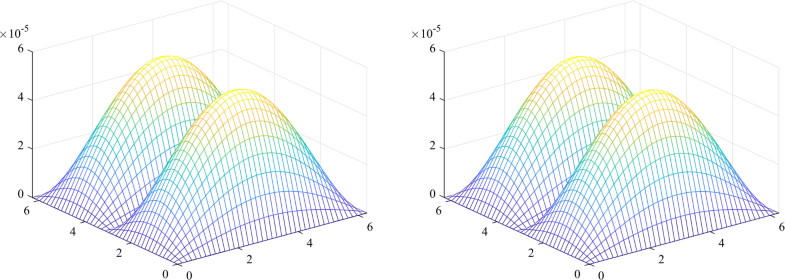Figure 12 The error of CFDS6-SSM (left-hand side) and E-CFDS6-SSM (right-hand side) when $$J=41$$, $$z=0.4\pi$$ and $$t=2$$ for $$\mathrm{SP} _{4}$$Figure 13 The error between the solution of CFDS6-SSM and solution of E-CFDS6-SSM when $$J=41$$, $$z=0.4\pi$$ and $$t=2$$ for $$\mathrm{SP} _{4}$$
Table 7

The convergence order and computational time for $$\mathrm {SP} _{4}$$ at $$t=2$$

Nodes

21 × 21 × 21

41 × 41 × 41

81 × 81 × 81

CFDS6-SSM

Max (error)

2.7607e−04

4.5042e−06

7.1814e−08

Time (s)

10.010231

40.045140

89.493375

Order

5.937

5.971

E-CFDS6-SSM

Max (error)

7.7141e−04

4.2592e−06

7.1633e−08

Time (s)

5.005856

10.031937

22.965542

Order

7.501

5.894

### Example 5

Consider the following 3D parabolic equation ($$\mathrm{SP} _{5}$$):
$$\textstyle\begin{cases} \frac{{\partial u}}{{\partial t}} - ( \frac{{{\partial ^{2}}u}}{{\partial{x^{2}}}} + \frac{{{\partial^{2}}u}}{{\partial{y^{2}}}}+\frac {{{\partial^{2}}u}}{{\partial{z^{2}}}} ) = {e^{ - t}} ( 3{\pi^{2}} - 1 )\sin\pi x\sin\pi y\sin \pi z,\quad (x,y,z) \in\varOmega, 0 < t \le1, \\ u(x,y,z,0) = \sin\pi x\sin\pi y\sin\pi z,\quad (x,y,z) \in\varOmega, \\ u(x,y,z,t) = 0,\quad (x,y,z) \in\partial\varOmega, 0 < t \le1, \end{cases}$$
where $$\varOmega= \{ (x,y,z); - 1 \le x \le1, - 1 \le y \le1, - 1 \le z \le1\}$$, ∂Ω denotes the boundary of Ω. The exact solution is $$u(x,y,z,t) = {e^{ - t}}\sin\pi x\sin\pi y \sin\pi z$$.
In this example, we observe the three-dimensional parabolic problem by the slice figures of the four-dimensional images in Fig. 14. In Fig. 14, the solutions of CFDS6-SSM and E-CFDS6-SSM are almost similar. In order to make the image more aestheticcally pleasing, we let $$z=-0.4$$, the figure becomes the three-dimensional figure in Fig. 15. We also list the absolute error of E-CFDS6-SSM and CFDS6-SSM in Table 8. It is easy to see that the results of E-CFDS6-SSM are in very good agreement with those of CFDS6-SSM, which means that the E-CFDS6-SSM without missing significant loss in accuracy. Then the corresponding error of CFDS6-SSM and E-CFDS6-SSM are drawn in Fig. 16. It can be seen that the CFDS6-SSM is slightly less accurate than E-CFDS6-SSM. In Fig. 17, the error between E-CFDS6-SSM and CFDS6-SSM is given. Besides, in Table 8, we also gave the computational time and order of convergence obtained by E-CFDS6-SSM and CFDS6-SSM, which indicates that the E-CFDS6-SSM is more efficient than the CFDS6-SSM for solving the parabolic equation and E-CFDS6-SSM holds same accuracy.Figure 14 The numerical solution of CFDS6-SSM (left-hand side) and E-CFDS6-SSM (right-hand side) when $$J=41$$ and $$t=1$$ for $$\mathrm{SP} _{5}$$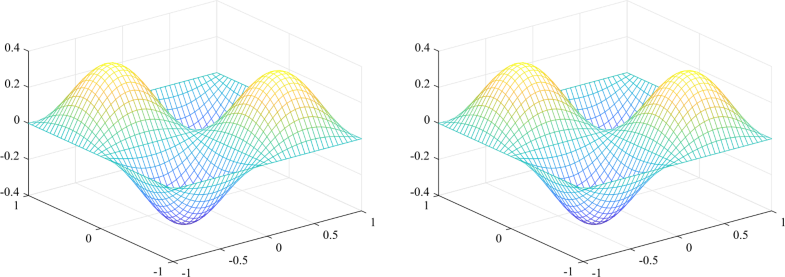Figure 15 The numerical solution of CFDS6-SSM (left-hand side) and E-CFDS6-SSM (right-hand side) when $$J=41$$, $$z=-0.4$$ and $$t=1$$ for $$\mathrm{SP} _{5}$$Figure 16 The error of CFDS6-SSM (left-hand side) and E-CFDS6-SSM (right-hand side) when $$J=41$$, $$z=-0.4$$ and $$t=1$$ for $$\mathrm{SP} _{5}$$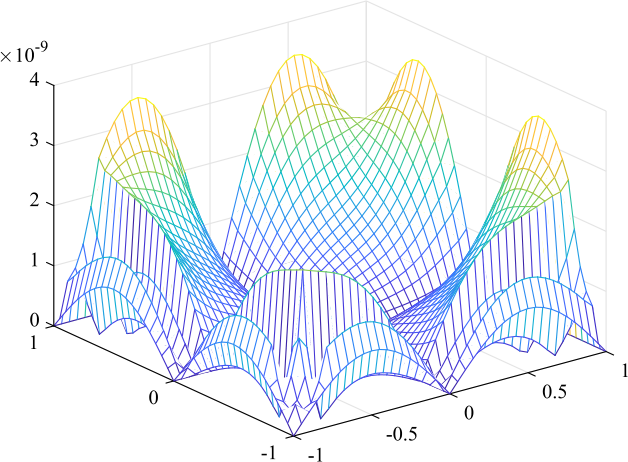Figure 17 The error between the solution of CFDS6-SSM and solution of E-CFDS6-SSM when $$J=41$$, $$z=-0.4$$ and $$t=1$$ for $$\mathrm{SP} _{5}$$
Table 8

The convergence order and computational time for $$\mathrm {SP} _{5}$$ at $$t=2$$

Nodes

11 × 11 × 11

21 × 21 × 21

41 × 41 × 41

CFDS6-SSM

Max (error)

0.0021

6.3364e−06

1.1076e−07

Time (s)

5.139907

121.805555

351.633034

Order

8.372

5.838

E-CFDS6-SSM

Max (error)

3.6402e−04

4.0606e−06

1.1199e−07

Time (s)

1.008504

24.672396

56.174196

Order

6.486

5.761

### Example 6

Consider the following 3D parabolic equation ($$\mathrm{SP} _{6}$$):
$$\textstyle\begin{cases} \frac{{\partial u}}{{\partial t}} - ( \frac{{{\partial ^{2}}u}}{{\partial{x^{2}}}} + \frac{{{\partial^{2}}u}}{{\partial{y^{2}}}}+\frac {{{\partial^{2}}u}}{{\partial{z^{2}}}} ) = 2{e^{ - t}}\sin x\sin y\sin z,\quad (x,y,z) \in\varOmega, 0 < t \le1, \\ u(x,y,z,0) = \sin x\sin y\sin z,\quad (x,y,z) \in\varOmega, \\ u(x,y,z,t) = 0,\quad (x,y,z) \in\partial\varOmega, 0 < t \le1, \end{cases}$$
where $$\varOmega= \{ (x,y,z);0 \le x \le2\pi,0 \le y \le2\pi,0 \le z \le2\pi\}$$, ∂Ω denotes the boundary of Ω. The exact solution is $$u(x,y,t) = {e^{ - t}}\sin x\sin y \sin z$$.
In this example, in Fig. 18, the numerical solutions of CFDS6-SSM and E-CFDS6-SSM are almost identical. Similarly, we let $$z=0.4\pi$$, the figure becomes the three-dimensional figure in Fig. 19. We list the absolute error and computational time of E-CFDS6-SSM and CFDS6-SSM in Table 9. It is easy to see that the results of E-CFDS6-SSM are in very good agreement with those of CFDS6-SSM, which means that the E-CFDS6-SSM does not have a significant loss in accuracy. It also shows that the E-CFDS6-SSM can greatly save the time consumption and vastly improve the computational time. Then we plot the numerical error and point-wise absolute errors of CFDS6-SSM and E-CFDS6-SSM in Figs. 20 and 21. It can be seen that the E-CFDS6-SSM is similar to CFDS6-SSM. Besides, in Table 9, we also give the order of convergence obtained by E-CFDS6-SSM and CFDS6-SSM, which indicates that the E-CFDS6-SSM is not only more efficient than the CFDS6-SSM for solving the parabolic equation, but it holds the same accuracy.Figure 18 The numerical solution of CFDS6-SSM (left-hand side) and E-CFDS6-SSM (right-hand side) when $$J=41$$ and $$t=1$$ for $$\mathrm{SP} _{6}$$Figure 19 The numerical solution of CFDS6-SSM (left-hand side) and E-CFDS6-SSM (right-hand side) when $$J=41$$, $$z=0.4\pi$$ and $$t=1$$ for $$\mathrm{SP} _{6}$$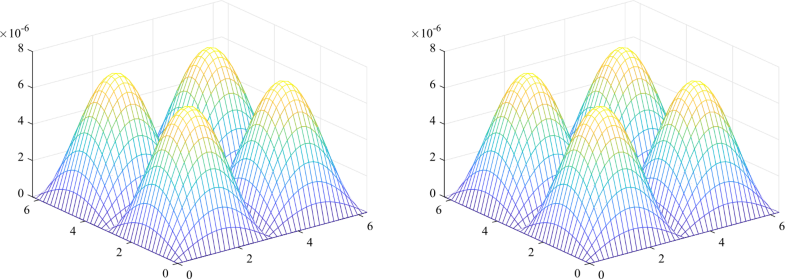Figure 20 The error of CFDS6-SSM (left-hand side) and E-CFDS6-SSM (right-hand side) when $$J=41$$, $$z=0.4\pi$$ and $$t=1$$ for $$\mathrm{SP} _{6}$$Figure 21 The error between the solution of CFDS6-SSM and solution of E-CFDS6-SSM when $$J=41$$, $$z=0.4\pi$$ and $$t=1$$ for $$\mathrm{SP} _{6}$$
Table 9

The convergence order and computational time for $$\mathrm {SP} _{6}$$ at $$t=1$$

Nodes

21 × 21 × 21

41 × 41 × 41

81 × 81 × 81

CFDS6-SSM

Max (error)

3.9145e−04

7.0154e−06

1.1455e−07

Time (s)

20.035465

45.750996

90.070817

Order

5.802

5.936

E-CFDS6-SSM

Max (error)

3.9128e−04

7.0141e−06

1.1455e−07

Time (s)

5.032226

10.448344

23.028154

Order

5.801

5.936

## 5 Conclusions

In this article, we have established the E-CFDS6-SSM for the numerical solution of MDPE. For this purpose, firstly, the snapshot is formed by means of the initial few solutions such as the numerical simulation results or the collection of experiment data and the POD basis is formulated by the SVD. Secondly, we have established E-CFDS6 based on the POD technique, which can be used to solve ODPE efficiently. Finally, we split MDPE into a product of ODPEs. Coupled with SSM, we have obtained the E-CFDS6-SSM for MDPE. As listed in the table of Sect. 4, we have compared our method with ADI, which shows that the E-CFDS6-SSM improves the accuracy greatly. In addition, the computational time of E-CFDS6-SSM is greatly less than CFDS6-SSM and the whole process of implementation of E-CFDS6-SSM is simpler than CFDS6-SSM. We test our algorithm by six numerical experiments, which implies that the E-CFDS6-SSM has high efficiency and is reliable for solving the MDPE.

## References

1. 1.
Hammad, D.A., El-Azab, M.S.: 2N order compact finite difference scheme with collocation method for solving the generalized Burgers–Huxley and Burgers–Fisher equations. Appl. Math. Comput. 258, 296–311 (2015)
2. 2.
Wang, H., Zhang, Y., Ma, X., Qiu, J., Liang, Y.: An efficient implementation of fourth-order compact finite difference scheme for Poisson equation with Dirichlet boundary conditions. Comput. Math. Appl. 71, 1843–1860 (2016)
3. 3.
Mohebbi, A., Abbaszadeh, M., Dehghan, M.: Compact finite difference scheme and RBF meshless approach for solving 2D Rayleigh–Stokes problem for a heated generalized second grade fluid with fractional derivatives. Comput. Methods Appl. Mech. Eng. 264, 163–177 (2013)
4. 4.
Düring, B., Fournié, M., Heuer, C.: High-order compact finite difference schemes for option pricing in stochastic volatility models on non-uniform grids. J. Comput. Appl. Math. 271, 247–266 (2014)
5. 5.
Chen, J., Ge, Y.: High order locally one-dimensional methods for solving two-dimensional parabolic equations. Adv. Differ. Equ. 2018, 361 (2018)
6. 6.
Bhatt, H.P., Khaliq, A.Q.M.: Fourth-order compact schemes for the numerical simulation of coupled Burgers’ equation. Comput. Phys. Commun. 200, 117–138 (2016)
7. 7.
Sari, M., Gürarslan, G.: A sixth-order compact finite difference scheme to the numerical solutions of Burgers’ equation. Appl. Math. Comput. 208, 475–483 (2009)
8. 8.
Zhang, X., Zhang, P.: A reduced high-order compact finite difference scheme based on proper orthogonal decomposition technique for KdV equation. Appl. Math. Comput. 339, 535–545 (2018)
9. 9.
Liang, Y.C., Lee, H.P., Lim, S.P., Lin, W.Z., Lee, K.H., Wu, C.G.: Proper orthogonal decomposition and its applications—Part I: theory. J. Sound Vib. 252, 527–544 (2002)
10. 10.
Rathinam, M., Petzold, L.R.: A new look at proper orthogonal decomposition. SIAM J. Numer. Anal. 41, 1893–1925 (2003)
11. 11.
Kerschen, G., Golinval, J.-C., Vakakis, A.F., Bergman, L.A.: The method of proper orthogonal decomposition for dynamical characterization and order reduction of mechanical systems: an overview. Nonlinear Dyn. 41, 147–169 (2005)
12. 12.
Ullmann, S., Rotkvic, M., Lang, J.: POD-Galerkin reduced-order modeling with adaptive finite element snapshots. J. Comput. Phys. 325, 244–258 (2016)
13. 13.
Dehghan, M., Abbaszadeh, M.: A combination of proper orthogonal decomposition–discrete empirical interpolation method (POD-DEIM) and meshless local RBF-DQ approach for prevention of groundwater contamination. Comput. Math. Appl. 75, 1390–1412 (2018)
14. 14.
An, J., Luo, Z., Li, H., Sun, P.: Reduced-order extrapolation spectral-finite difference scheme based on POD method and error estimation for three-dimensional parabolic equation. Front. Math. China 10, 1025–1040 (2015)
15. 15.
Luo, Z., Li, H., Sun, P.: A reduced-order Crank–Nicolson finite volume element formulation based on POD method for parabolic equations. Appl. Math. Comput. 219, 5887–5900 (2013)
16. 16.
Peaceman, D.W., Rachford, H.H. Jr.: The numerical solution of parabolic and elliptic differential equations. J. Soc. Ind. Appl. Math. 3, 28–41 (1955)
17. 17.
Seydaoğlu, M.: An accurate approximation algorithm for Burgers’ equation in the presence of small viscosity. J. Comput. Appl. Math. 344, 473–481 (2018)
18. 18.
Gidey, H.H., Reddy, B.D.: Operator-splitting methods for the 2D convective Cahn–Hilliard equation. Comput. Math. Appl. 77, 3128–3153 (2019)
19. 19.
Sun, J., Eichholz, J.A.: Splitting methods for differential approximations of the radiative transfer equation. Appl. Math. Comput. 322, 140–150 (2018)
20. 20.
Einkemmer, L., Moccaldi, M., Ostermann, A.: Efficient boundary corrected Strang splitting. Appl. Math. Comput. 332, 76–89 (2018)
21. 21.
Li, J., Chen, Y., Liu, G.: High-order compact ADI methods for parabolic equations. Comput. Math. Appl. 52, 1343–1356 (2006)
22. 22.
Li, J., Visbal, M.R.: High-order compact schemes for nonlinear dispersive waves. J. Sci. Comput. 26, 1–23 (2006)
23. 23.
Li, J.: High-order finite difference schemes for differential equations containing higher derivatives. Appl. Math. Comput. 171, 1157–1176 (2005)
24. 24.
Sun, P., Luo, Z., Zhou, Y.: Some reduced finite difference schemes based on a proper orthogonal decomposition technique for parabolic equations. Appl. Numer. Math. 60, 154–164 (2010)
25. 25.
Luo, Z., Yang, X., Zhou, Y.: A reduced finite difference scheme based on singular value decomposition and proper orthogonal decomposition for Burgers equation. J. Comput. Appl. Math. 229, 97–107 (2009)
26. 26.
Luo, Z., Li, H., Sun, P., Gao, J.: A reduced-order finite difference extrapolation algorithm based on POD technique for the non-stationary Navier–Stokes equations. Appl. Math. Model. 37, 5464–5473 (2013)

## Copyright information

© The Author(s) 2019

Open Access This article is distributed under the terms of the Creative Commons Attribution 4.0 International License (http://creativecommons.org/licenses/by/4.0/), which permits unrestricted use, distribution, and reproduction in any medium, provided you give appropriate credit to the original author(s) and the source, provide a link to the Creative Commons license, and indicate if changes were made.

## Authors and Affiliations

• Baozou Xu
• 1
• Xiaohua Zhang
• 1
• 2
1. 1.College of ScienceChina Three Gorges UniversityYichangChina
2. 2.Three Gorges Mathematical Research CenterChina Three Gorges UniversityYichangChina

## Personalised recommendations